﻿ Singlet, Triplet, anomalous Zeeman effect in Spin

Singlet, triplet, anomalous Zeeman don't mean "Spin".

"Spin" is an illusion, again.

(Fig.1) Electron spin ? How ? Illusion ?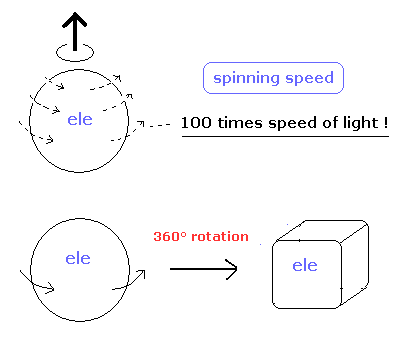"Spin" is one of the most important and fundamental concepts in the current quantum theory.
"Spin" is indispensable for Pauli exclusion principle, entanglement, fermi statistics, particle physics and string theory.

But strange to say, if you try to ask some specialists in these fields about what "spin" really is, they cannot give clear picture about spin at all.
It's very strange, though as many as 100 years have passed since quantum mechanics and "spin" were born.

Because as shown on this page, "spin" cannot be described using real objects.
Even if you rotate spin 1/2 particle by 360 degree, it cannot return to its original configuration.
And a single electron is a point-like particle with small mass, its spinning speed must exceed the light speed by far.

(Fig.2) Point particle → spinning speed is much faster than light !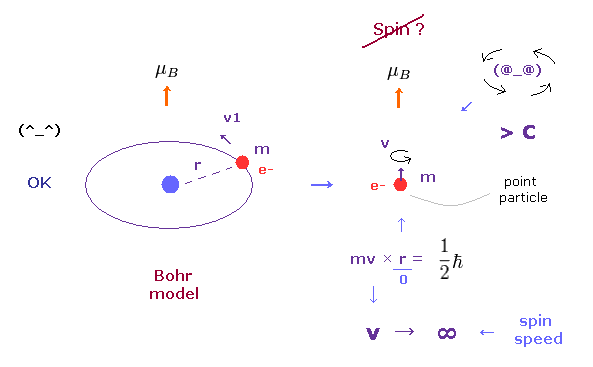In the ground state hydrogen atom, the magnetic moment of an electron is known as Bohr magneton (= μB ).
In Bohr model, this magnetic moment is generated by usual circular movement of an electron.
But as quantum mechanics lacks angular momentum ( L = 0 ) and circular movement, this magnetic moment μB is caused by "spin", they insist. ( See also this page. )

As you see in Fig.2, it is impossible that point-like particle electron generates angular momentum 1/2 ħ.
Angular momentum is given by mv×r, where radius "r" is almost zero in point particle.
As a result, rotating speed "v" must be much faster than light to reach 1/2 ħ.

(Fig.2') Proton's spin OK.   Electron spin is illusion !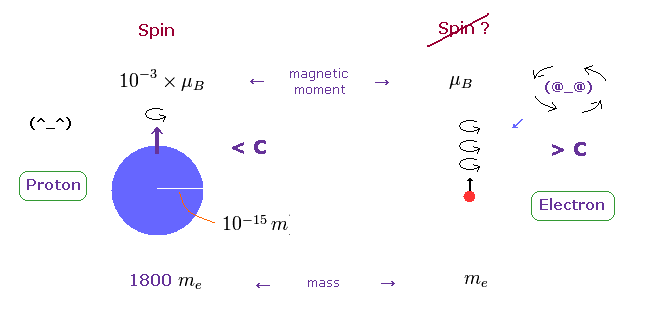On the other hand, heavy proton with some size can produce "real" spin, NOT exceeding light speed.
So nuclear spin is real, and electron spin is an illusion, it's only circular orbit like Bohr model.

In fact, the magnetic moment of electron spin is about 1000 times bigger than that of proton !
This wide discrepancy indicates electron spin is rotating in circular orbit with some radius, NOT on its own axis.

Origin of Pauli exclusion principle ---- Not "spin".

[ Spin magnetic moment is too weak to cause Pauli exclusion principle. ]

(Fig.3) Spin really causes ferromagnetism and Pauli exclusion ?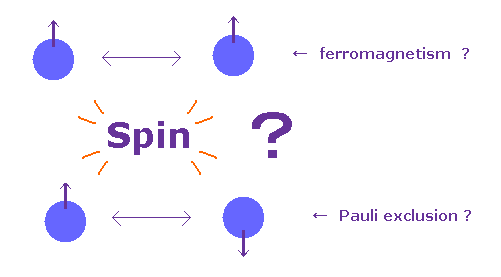In textbooks, they say each spin of atom tries to be parallel to each other in ferromagnetism.
And in Pauli exclusion principle, the third spin cannot enter the same orbital, where "up" and "down" spin particles already exist.

This means spin magnetic moment (= up or down ) has the power enough to cause these phenomena ?
In fact, spin magnetic moment is too weak to cause these ferromagnetism and Pauli exclusion principle, because the magnitude of spin-spin interaction is extremely small, only fine structure level (= 1.0 × 10-5 eV ).

(Fig.4) Repulsive force of Pauli exclusion principle is very strong (= about 11 eV in Li ).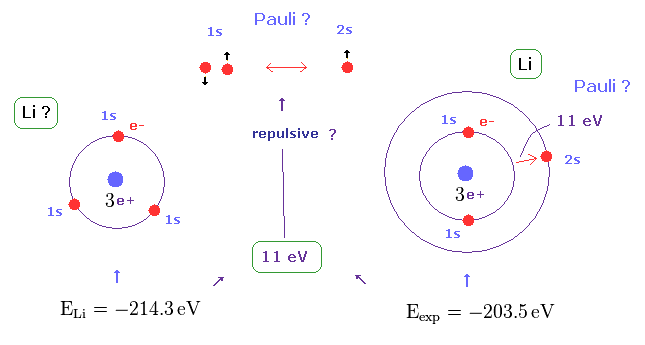According to this book ( Quantum chemistry 6th edition, by Ira N. Levine, p 292 ), the ground state energy of lithium becomes lower than -214.3 eV, if all three electrons enter 1s orbitals.
On the other hand, the experimental value of actual lithium is -203.5 eV in which one of three electrons enters 2s ( NOT 1s ) orbital.

This means the third electron is expelled from 1s orbital by some force, though Coulomb attraction of nucleus is strong enough.
This mysterious repulsive force of about 11 eV is due to Pauli exclusion principle, as shown in Fig.4.

(Fig.5) Spin-Spin interaction is too weak.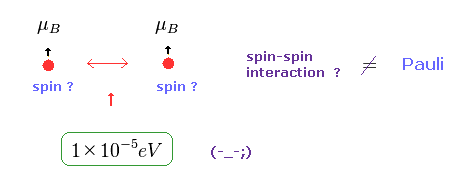In Pauli exclusion principle, "spin" direction is crucial factor for deciding whether each electron enters the same orbital or not.
But magnetic dipole interaction of spin is basically too weak (= about fine structure level, 1 × 10-5 eV. ), which can NEVER reach as much as 11 eV.

This means we need other concepts to describe this strong repulsive force of Pauli exclusion.
If "spin" ( magnetic moment ) is too weak, there is only one thing left.
As shown on this page, de Broglie wave ( destructive ) interference is the main generator of this Pauli repulsive force.

Ferromagnetism and diamagnetism are NOT "spin".

(Fig.6) Origin of ferromagnetism = Spin ??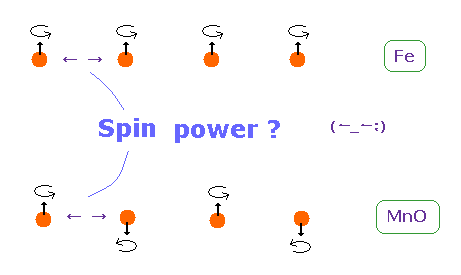In ferromagnetism, they insist "spin" of each atom such as Fe tends to be parallel to each other.
On the other hand, in antiferromagnetism ( or diamagnetism ) such as MnO, each spin tends to be antiparallel to each other.

So the origin of (anti) ferromagnetism is "spin", as most textbooks say ??
In fact, spin magnetic ( dipole ) moment interaction is too weak to cause (anti) ferromagnetism.

(Fig.7) Origin of ferromagnetism = Exchange interaction "J" ??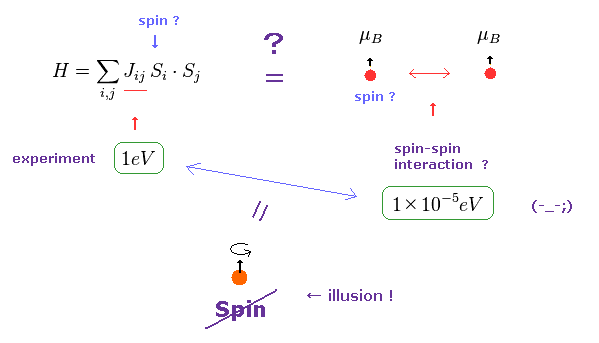In the current quantum theory, they describe the force of (anti) ferromagnetism as coupling constant (= J ) in Heisenberg model.
The magnitude of coupling constant (= exchange interaction ) is as big as 1 eV, which is much stronger than spin magnetic dipole interaction (= 10-5 eV ).

Spin magnetic moment interaction is much too weak to account for most magnetic materials such as ferromagnet, as is referred to in various sites ( See this (p.7 or 8), this (p.5), this (p.20), this (p.7) ).
Spin dipole interaction is as small as 0.3 K as temperature expression, which is easily broken at room temperature.
( For example, iron can become ferromagnetism at 1043 K, which is much bigger than 0.3 K. )

(Fig.8) De Broglie wave interaction is the origin of ferromagnetism.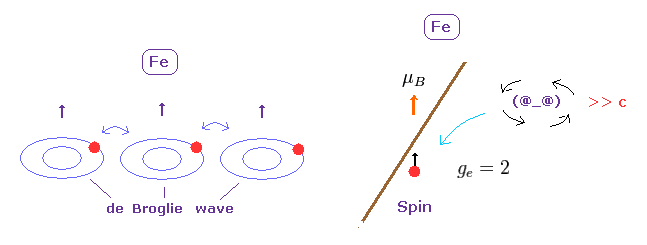Spin - spin magnetic interaction is unrealistically too weak to describe magnetic materials.
Again, there is only one thing left.

Like strong repulsive Pauli exclusion, it is quite natural that we think these synchronous de Broglie waves makes ferromagnetism stable at room temperature.
As shown in Davisson-Germer experiment, de Broglie wave interference has the power to change the direction of Coulomb force.

Singlet and Triplet cannot be caused by "spin".

(Fig.9) Singlet and Triplet are just "math" symbols.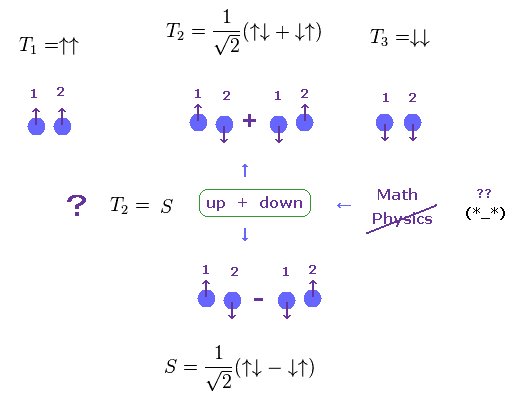It is said "spin" is the origin of singlet and triplet terms of atoms.
But in fact, the energy difference of these singlet and triplet levels is as much as Coulomb interaction, which cannot be explained by spin magnetic interaction. ( See Fig.10. )

Furthermore, these singlet and triplet states are completely different from real ones.
Both in T2 of triplet and singlet (=S ), one of two electrons is "up" spin, another is "down spin".
So these T2 and S are the same energy states ?
But they insist these states are different, using math symbol (= ± ).

These strange math symbols (= ± ) denoting up and down spins cannot be expressed using real physical objects.
As a result, singlet and triplet states are other things such as Coulomb interaction in multi-electron atoms, Not "spin".

(Fig.10) Singlet and Triplet are as strong as Coulomb interaction.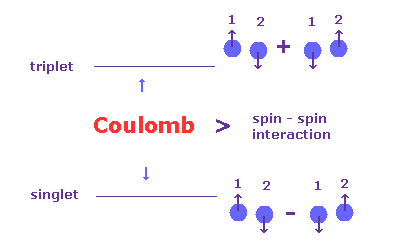In the book, Story of Spin ( S. Tomonaga ), they say singlet and triplet cannot be caused by spin-spin interaction.

----------------------------------
[ p.84 ] Now what is the order of magnitude of the spin-spin interaction Es,s ?
We can answer this from the experimental data given in figure 5.2 by compareing the levels of the singlet and triplets terms. ---- Then we find that the interaction between spins is quite large.

[ p.85 ] However such energy of "magnetic" origin causes a difference between (n, l, 0) and (n, l, 1) of only the order of magnitude of splittings seen in alkali doublet (= fine structure ) levels.
On the contrary, from figure 5.2 we see that the difference is as big as that of "electric" origin.
This was an enigma for many years.
-------------------------------------

Anomalous Zeeman effect of hydrogen does NOT mean "spin".

(Fig.11) Anomalous Zeeman effect of H atom ?   ↓ = Bohr-Sommerfeld model.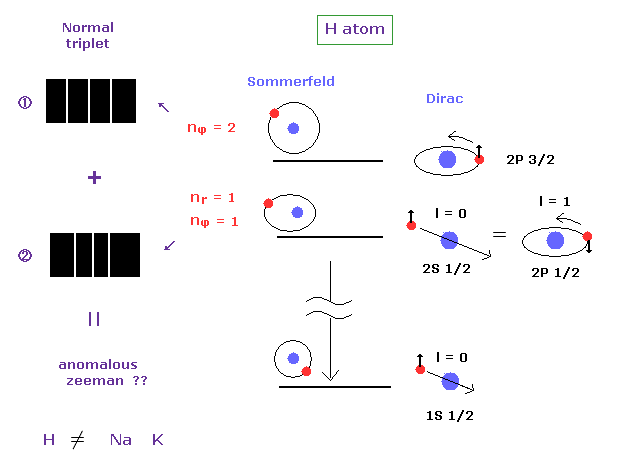It is known that the spectrum lines in hydrogen atom is much fewer than other alkali atoms such as sodium and potassium.
If we suppose electron "spin", energy levels of H atom must be much more spilt.
So they came to insist various levels of H atom accidentally agree with each other ( 2s1/2 = 1p1/2 ... ), as shown on this page.

In the definition of normal Zeeman effect, each "n" energy level should have singlet ( NOT doublet ) term, and is split into triplet under magnetic field.
Most websites ( and books ) say H atom also exhibits anomalous Zeeman effect.
But this anomalous Zeeman of hydrogen can be explained by Sommerfeld's fine structure.
In H atom, each fine structure doublet is split into ( normal ) triplet ( 2 × 3 = 6 ).

(Fig.12) J Mehra's book.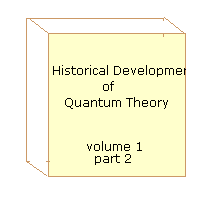On page 659 of the Historical Development of Quantum Theory v.1 part2 ( J. Mehra ).
--------------------------------
In 1922, Otto Oldenberg obtained the following results :
For weak fields, each component of Balmer Hα doublet was separated in a normal Zeeman triplet, but for strong fields, the Zeeman patterns of the doublet changed into the Zeeman pattern of a singlet line.
---------------------------------
They just call these doublets "anomalous Zeeman effect" in hydrogen atom.
But as you know, these doublets (= fine structure ) can be completely explained by Bohr-Sommerfeld model, Not depending on strange "spin".

(Fig.13) History of Zeeman effect.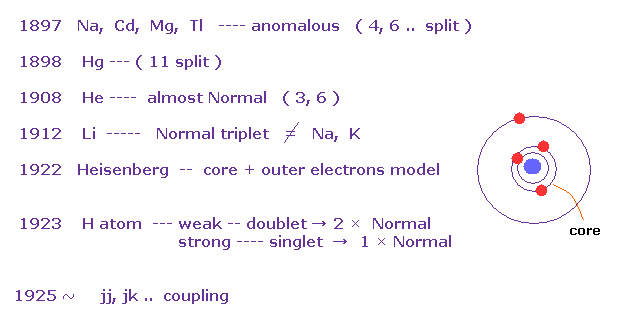According to the above book, anomalous Zeeman effects are often seen in multi-electron atoms such as Na, Cd, Mg..
In mercury (= Hg ), as much as 11 split lines were observed under magnetic fields in 1898.

The important point is that they didn't mention hydrogen atom, when they say about anomalous Zeeman effect ( Fig.11 is the only exception ).
And this book also say most multi-electron atoms ( excluding light atoms H, He, Li ) exhibit much more complex lines than simple doublet and triplets.

As a result, Heisenberg and Lande thought anomalous Zeeman effect is caused by core electrons, Not spin, at first.
And various atoms and metals do NOT obey Lande g-factor.
This fact made Russell and Saunders create new concepts such as jj coupling and jk coupling.

(Fig.14) Normal Zeeman.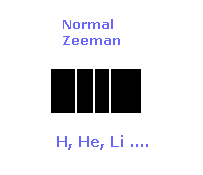Light atoms such as lithium and helium are usually NOT mentioned as an example of anomalous Zeeman effect.
On page 450 of the above book, they say " the red lithium line had NOT been separated so far. They found a triplet, which surprised them very much.
Because lithium is clearly different from sodium and potassium cases.

After that, they came to insist lithium atom exhibits Paschen-Back (= almost normal Zeeman ) effect.
As electrons of atoms increase in number, more separated lines are observed.
This tendency clearly shows anomalous Zeeman effect is caused by interactions with other electrons, Not "spin".

Quantum spin is unreasonable.

(Fig.15) Spin magnitude is NOT "1/2" according to quantum mechanics ?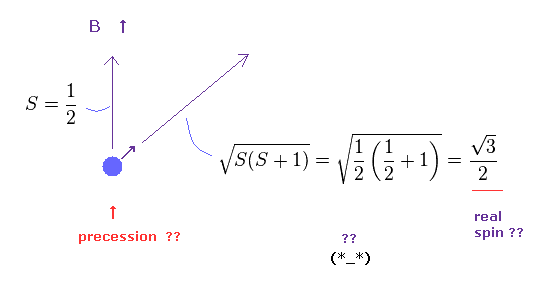Under the magneic field, we can observe Bohr magneton as spin magnetic moment.
This means spin anglar momentum S = ± 1/2 in the direction of magnetic field (= B ).
Strange to say, according to quantum mechanics, true spin anglular momentum is bigger than 1/2, as shown in Fig.15
True magnitude of angular momentum must be expressed using "square root" due to spin precession, they insist.

(Fig.16) Spin + Spin ?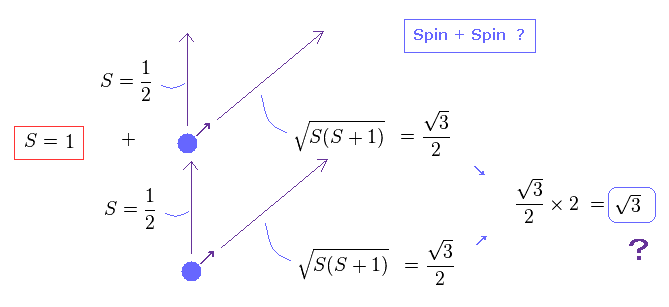The problem happen when we think about the sum of spins in multi-electron atoms.
They just simply sum up spins of all outer electrons as total spin effect.
So when two electrons exist, total spin in the direction of magnetic field becomes 1/2 + 1/2 = 1, they insist.
As a result, the true magnitude ( in the direction of precession ) of their total spin becomes a square root of 3, as shown in Fig.16 ?

(Fig.17) Spin + Spin ← self-contradiction !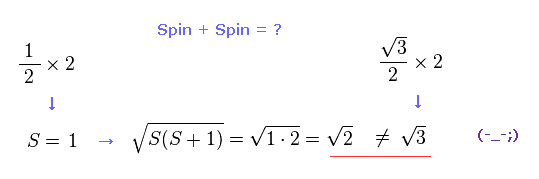But when you subsitute S = 1 into the equaiotn of a square root of S(S+1), this result becomes a square root of 2, ( NOT 3 ! ).
This is completely inconsistent with the result of Fig.16 and Fig.15, and self-contradiction.

So when two electrons exist in two ( different ) outer orbitals, they are fine-tuning precession states of each other so that the total spin becomes just a square root of 2 ? Impossible !
As you feel, these quantum interpretation about spin is very unnatural and unrealistic.

(Fig.18) Lande g factor is real ?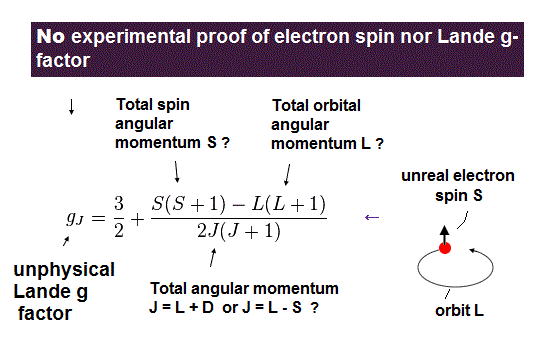As shown on this page, Lande g factor is based on very unnatural precessions.
In multi-electron atom, we need to sum up each spin like Fig.18, they insist.
As a result, the self-contradiction of Fig.16 and Fig.17 occurs.

(Fig.19) Observed magneton in the direction of magnetic field H.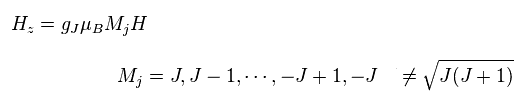Using Lande g factor of Fig.18, Hamiltonian of observed atomic magneton in the direction of magnetic field H becomes like Fig.19.
The important point is that quantum number (= Mj ) in z direction is an integer, Not a "square root" of J(J+1).

(Fig.20) Observed magneton in the direction of magnetic field H.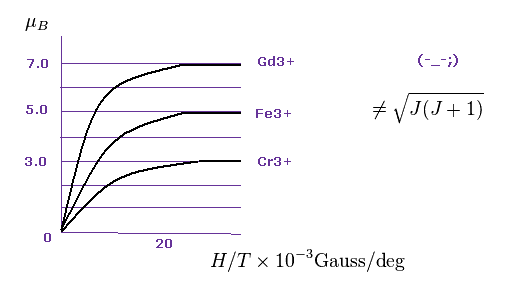Data of metallic ion magnetic moments such as Gd2+, Fe3+ and Cr3+ are seen in this paper ( W.E. Henry, Phys. Rev. 88 559, 1952 ).
As shown in Fig.20, each saturation magnetization becomes an integer times Bohr magneton (= μB ).
Gadolinium ion is 7.0 × μB.
Iron ion is 5.0 × μB.   Chromium ion is 3.0 × μB.
And oxygen molecule shows 2.0 × μB ( P.Curie, 1895 ).

So Bohr-Sommerfeld quantization holds in these atoms.

(Fig.21)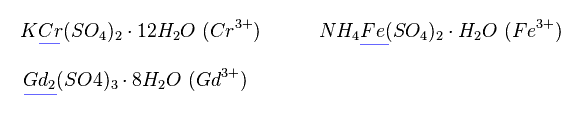Magnetic moment of these ions cannot be measured individually.
They measure the states of compounds like Fig.21.
So when the influences by other atoms are strong, magnetic moment becomes more complex.
Actually, many neutral metals such as Fe, Cr and Gd cannot be described using Lande g factor.
Effects by other atoms are big, they insist.

(Fig.22)Cr3+ has three outer electrons in 3d orbitals.
According to Hunt law, total angular momentum L and spin S become "3" and "3/2" in Cr3+.
As I said above, very weak spin magnetic moment has NO power to make them all parallel.
Do you think the rule of Fig.22 is too simple and too good to be true ?

[ Lande g factor is violated in various transition metals. ]

(Fig.23) Cr3+ ion disobeys Lande g factor.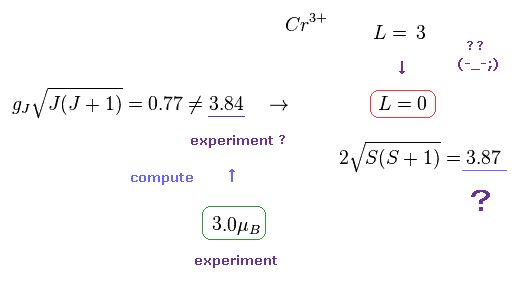In fact, transition metals such as Ti3+, V3+, Cr3+, Fe2+, Co2+, Ni2+, and Cu2+ do NOT obey Lande g factor.
Strange to say, the total angular momentum (= L ) of these ions becomes completely zero, they insist.

Though the angular momentum of Cr3+ in Hunt law is L = "3", this L becomes "0" by the influence of surrounding atoms, they insist. Do you think these interpretations are inconsiderate and too good to be true ?

In most textbooks, they tend to use effective Bohr magnetic number, which is expressed as a square root of J(J+1).
But as I said in Fig.20, these J(J+1) and S(S+1) are NOT observed values.
From intergers gotten from experiment, they only calculate a squre root in each effetive number.
So all of these transition metallic ions show an integer times Bohr magneton like Bohr model.

(Fig.24) Gd3+ ion also shows an integer times Bohr magneton.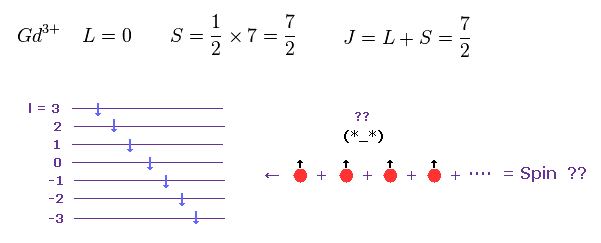According to Hunt law, total angular momentum and spin of Gd3+ become like Fig.24.
Each spin belongs to different orbital.
So it is very unnatural that total spin angular momentum of "7" spins becomes just S = 7/2, though each electron is separated from each other.

(Fig.25) Gd3+ ion also shows an integer times Bohr magneton.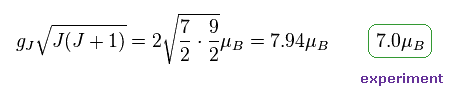In most textbooks, effective Bohr magnetic number "7.94" is shown as Gd3+ ion.
But as I said in Fig.20. observed magnetic number becomes an integer times Bohr magneton also in Gd3+.
They just calculate 7.94 based on 7.0.
Square root of J(J+1) itself means unrealistic "precession", which cannot be observed directly.

Magnetic models are just math symbols, NOT physics.

(Fig.26) "Spin" is just math symbols ?Fig.26 is Heisenberg spin model, which is often used even in the current condensed matter physics.
But this model is too abstract to describe various complicated and dynamic phenomena.
Depending on whether J is negative or positive, this atom becomes ferromagnet or antiferromagnet, they insist.

As you feel, it is impossible to express actual electron's complicated motion in various atoms, using only three simple math symbols (= J, S, S ).
They have given up asking what "spin" and Pauli exclusion principle really are, forever.
So all they can do is to rely on very abstract math symbols.
Parameter "J" must be determined from experiment ( NOT from theory ). They just try to fit model to actual data.

(Fig.27) XY model ?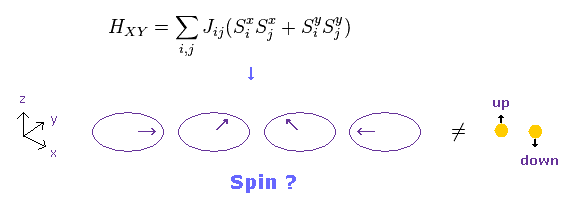Conveniently, they introduced "XY" model, in which each spin turns toward x or y directions.
If "x" and "y" spin directions are also allowed, the concept of "spin" becomes very doubtful.
Beucause this XY model is completely inconsistent with parallel and antiparallel rule of spins.

And when spin is in the x direction, there are three different spin states such as "up", "down" and "horizontal".
As a result, as many as three electrons can enter the same orbital.
It means Pauli exclusion principle breaks down. This is strange.

(Fig.28) XXZ model ?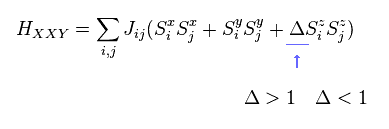Furthermore, in XXZ model, each spin can turn toward various directions (= x, y, z ! ).
Each parameters such as J and Δ need to be determined from experiment.
Because each metal contains almost infinte atoms, they cannot compute all effects by quantum chemistry.

They often use density functional theory (DFT) in condensed matter and band calculation.
Because DFT doesn't take so much time.
Instead, this DFT must use artificial approximations such LDA and various potentials to fit experimental results.
So these DFT is NOT ab-initio at all, though they like to call it "ab-initio DFT". This name is NOT ture.

(Fig.29) ↓ Spin-orbit interaction ?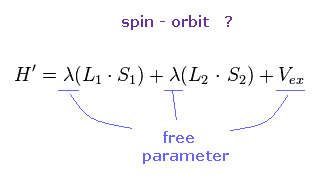Each metal contains various atoms, so it is impossible to estimate spin-orbit interaction without relying on some approximation and experimental results.
λ of Fig.29 is spin-orbit coupling constant, which cannot be determined only by theory itself.
Because this λ varies complexly depending on binding states and environment.

First, it is impossible to see unreal spin itself, so we cannot confirm spin-orbit interactions really exist
As I said above, tha magnitude of spin-orbit interaction is almost same as fine structure of Bohr Sommerfeld model.

Spin waves, magnon are "unreal" concepts.

[ Quasiparticle, magnon and spin wave don't really exist. ]

(Fig.30) Magnon, spin wave = "math" symbol, NOT physics.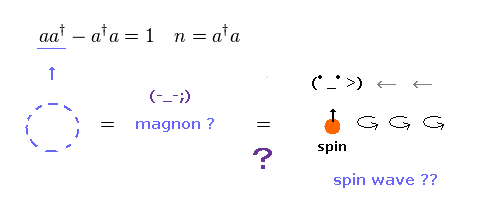Spin waves are said to be propagating in magnetic materials.
They are also called "magnon", which is a collective excitation of electrons' spin structure.
Of course, this magnon is unreal quasiparticle.
Magnon is "boson", which is just creation (= a ) and annihilation (= a ) "math" operators.

It is said that these spin waves can be detected by neutron scattering.
But there are many other factors influencing neutron's scattering and energy change.
So it is impossible to prove the existence of spin wave itself directly.
They only estimate their existence.
( Though "quasiparticle" itself does NOT really exist.... )

(Fig.31) Quasiparticle "magnon" causes spin wave ??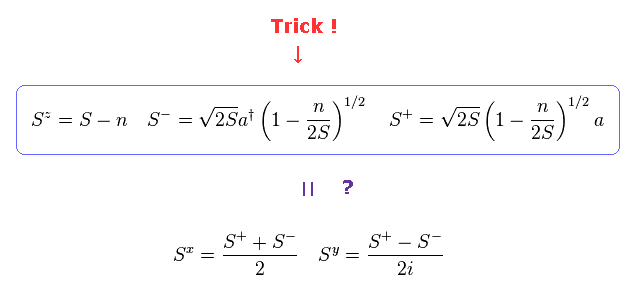Artificial transformation like Fig.31 is one of the main reasons why I often say "spin" is just math, NOT physics.
Using creation (= a ) and annihilation (= a ) operators of magnon (= Fig.30 ), they try to express "Spin" compoment (= S ), which is called Holstein-Primakoff transformation.

But as you see Fig.31, the forms of these transformations were artificially introduced by our human beings, and do NOT mean the truth of nature.

(Fig.32) Spin relations ?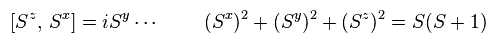If we use the transformation of Fig.31, we can derive spin relations like Fig.32.
So they insist, quasiparticle magnon causes "spin wave".
Can you think these abstract "math" operators really exist ?

(Fig.33) Spin Hamiltonian ?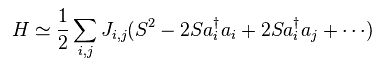Using this transformation in Heisenberg spin model of Fig.26 and do some approximations, we can get Hamiltonian of Fig.33.
Performing Fourier transformation of Fig.34 in Fig.33,
(Fig.34)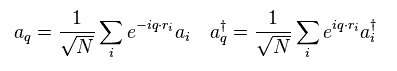we have

(Fig.35) Creation of magnon = Spin wave energy ??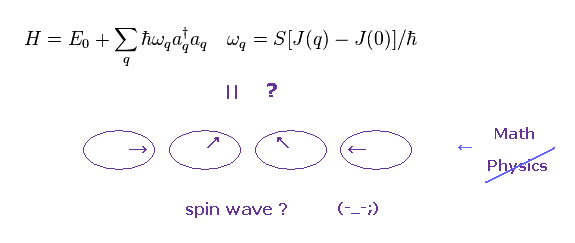In Fig.35, when quasiparticle magnon (= aa ) is created, spin wave is generated, they insist.
Of course, we cannot see these unreal quasiparticles and waves directly.

They just estimate these existences from some change of scattered neutrons. or lights.
Neutrons are said to be scattered by some magnetic moment, but there are various other factors influencing these scattering results.
So "spin wave" itself is only an imaginary thing.

Proof of artificial trick of spin.

In fact, even the latest experiments and theories dealing with "spin" are based on very artificial tricks.
There are good ( bad ) examples proving spin theories have NO reality in the page of Tricks in "Spin".

Sodium D lines = Spin-orbit interaction !?

(Fig.36) Sodium fine structure is too big to be explained by a single electron's spin.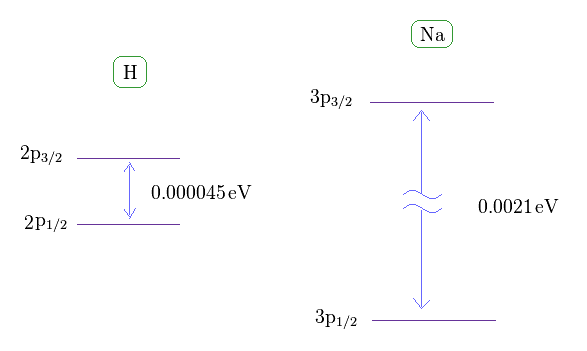As shown on this site, hydrogen fine structure (= doublet ) between 2p3/2 and 2p1/2 is about 0.000045 eV.
On the other hand, the fine structure between 3p3/2 and 3p1/2 of sodium is as big as 0.0021 eV, as shown on this site.

Approximately, we can consider the outer 3p ( or 3s ) electron of sodium is moving around Z = +1 central core charge.
( "Core" is the total charge of Na nucleus and all electrons contained in n = 1 and 2 orbits. )

From the viewpoint of this outer electron, the core charge is moving around in the opposite direction, which causes magnetic field at the point of the electron having "spin".
As a result, spin-orbit interaction is produced, they insist.

[ Sodium D line is caused by spin - orbit coupling ? → "Z" becomes too large ( 1 → 3.54 ). ]

(Fig.37) Core charge of sodium is much bigger than Z = 1 !?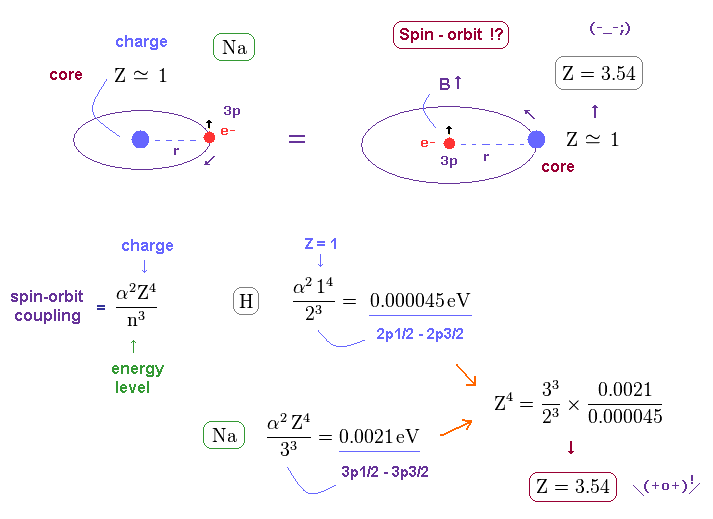In this section, we explain the reason why this big fine structue in sodium is very unreasonable with respect to spin-orbit interaction.
Both in hydrogen and sodium's outer (= 3p ) electron, the effective central (= core ) charges they feel are about Z = +1e.

But the discrepancy between these H and Na spin-orbital interactions are too wide.
This means the effective central charge in sodium is much bigger than Z = 1 (= about Z = 3.54 in Na ), which is very unreasonable and unrealistic. See also this page.

(Fig.38)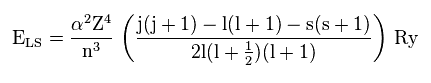As shown on this site (p.9), the energy by spin-orbit interaction becomes like Fig.38.
α and Ry are constants, so the principal quanum number (= n ), angular momentum (= l ), and central ( core ) charge (= Z ) are crucial factors for deciding this interactive energy.

(Fig.39)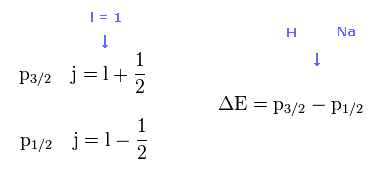Both in 2p (= H ) and 3p (= Na ) fine structures, the angular momentum (= l ) is equal to 1, so total angular momentum j = 1 ± 1/2, which are completely the same in H and Na.
So the difference between H and Na are only principal quantum number ( n = 2 or 3 ) and core charge ( Z = 1 or ? ). See also this site .

(Fig.40) Energy difference between p3/2 and p1/2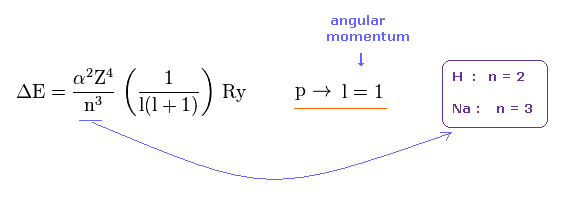Eq.40 shows the energy difference between p3/2 and p1/2.
Here α is fine structure constant, and Ry is Rydberg constant.
The principal quantum numbers (= n ) of Na and H are " 3 " and " 2 ", respectively
So the energy difference is smaller in Na, if core charge Z is the common ( Z = 1 ).

(Fig.41)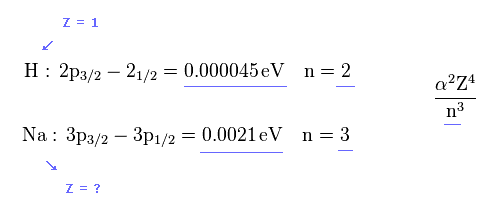But the energy difference of Na is much larger than H.
( 0.0021 / 0.000045 = 46.6 times bigger ).
So the effective core charge (= Z ) of Na must be much bigger than "1", which is strange.

(Fig.42) Effective "Z" becomes too big (= 3.5 ), if spin-orbit coupling is true.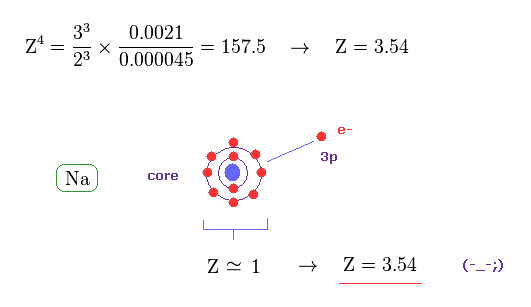From Eq.40 and Eq.41, the effective core charge of Na becomes as large as Z = 3.54, which is unrealistic.
This core charge is the total charge summing up Na nucleus and all 1n, 2n electrons.
The charge of Na+ ion ( excluding only 3s electron ) is about +1e.
So, this core charge "Z" must be close to "1" also in Na.

On page 144 of "Modern Atomic and Nuclear Physics" ( Fujita Yang, Joseph H.Hamilton ).
↓
-----------------------------------------------------------------------
However, calculation of the spilitting of the 3P level in sodium is a little difficult ...
Substituting the effective charge Zeff into Eq.4.42 (= Fig.40 ) to replace Z, and using experimentally measured value ΔU = 2.1 × 10-3 eV, we may calculate from Eq.4.42 ( n = 3, l = 1 ), Zeff = 3.5.
----------------------------------------------------------------------

In conclusion, it is very unnatural that we consider the fine structure of Na D lines is caused by spin-orbit interaction.
As far as I see various websites and textbooks, there are NO reasonable reason why the Na fine structure is much bigger than H.

(Fig.43) Coulomb and large (core) orbit - orbit interactions generate Na D lines.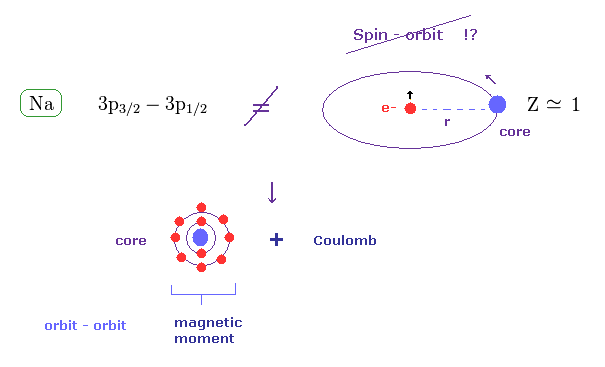In the present Lange g factor, they insist there are No magnetic moments in the inner (= core ) electrons in Na.
But from the realistic viewpoint, the inner shells contain various large orbital motions.

So we can naturally think the various Coulomb interaction and large magnetic moment of inner electrons cause this large fine structure in sodium D lines.
Spin- orbit interaction is too weak to explain sodium D lines.

(Fig.44)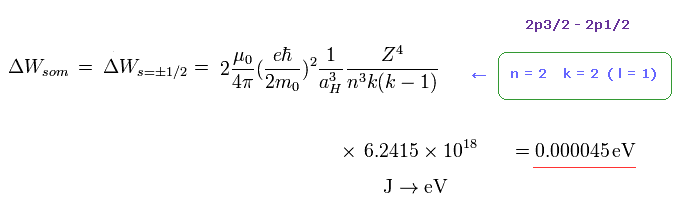As shown on this page or the book "Story of Spin" ( S. Tomonaga ), the fine structure by Bohr-Sommerfeld model is just equal to spin -orbit interaction using Thomas factor.
In Fig.44, " k = 2 " corresponds to "l = 1".

Substituting "n = 2" and " k = 2 " into Fig.44, the energy difference between 2p3/2 and 2p1/2 is just equal to 0.000045 eV (= experimental value ).
So the Bohr-Sommerfeld model can explain this fine structure correctly without relying on unrealistic "spin".

According to Fig.44, the energy difference between 3p and 3d is about 0.0000044 eV, which is close to Lamb shift
So very small Lamb shift can be explaied by various other factors, NOT by strange virtual particles and divergence.

[ How big is effective core charge Z of sodium from ionization energy ? ]

(Fig.45) What is effective core charge Z from ionization energy ?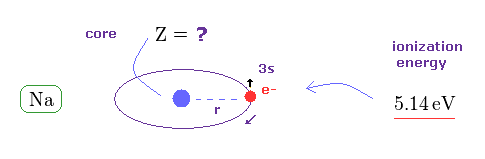In Fig.42, if spin-orbit interaction is real, effective core charge Z of sodium becomes much bigger (= 3.54 ) than "1".
We can know this effective core charge from ionization energy of sodium ( 3s ) outer electron.
This ionization energy of sodium is 5.14 eV.

(Fig.46)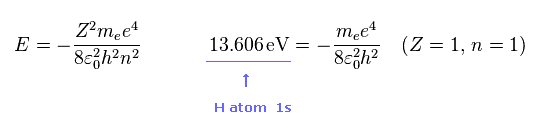As you know, hydrogen ionization energy of 1s electron is 13.606 eV.
In 1s hydrogen, Z = 1 and n = 1.

(Fig.47)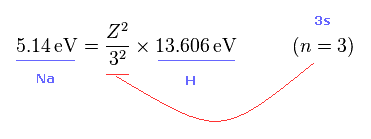From Fig.45 and Fig.46, we get the relation of Fig.47.
Z of Fig.47 means effective core charge from the viewpoint of Na 3s electron.

(Fig.48)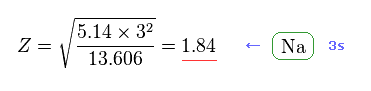From Fig.47, we get Z = 1.84 as the effective core charge of sodium.
This value (= 1.84 ) is completely different from 3.54 of Fig.42.

Due to gaps in 1s and 2s electron's shells, effective core charge which 3s electron feels becomes a little bigger than "1".
But "Z = 3.54" is too big and unrealistic.

(Fig.49)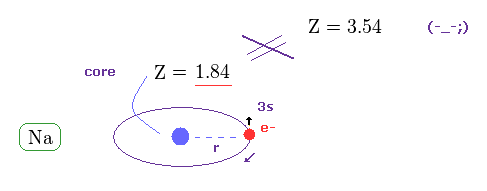This is clearly self-contradiction, and shows spin-orbit interaction of sodium D lines is wrong.
( Of course, 3p electron is more apart from Na nucleus, so effective core charge must become smaller than 1.84. )

Also in other alkali metals such as K, Rb, Cs, the concept of spin orbit coupling is violated.

Einstein-de Haas effects showed "Spin" is contradictory.

(Fig.50) Spin angular momentum can be macroscopically seen ?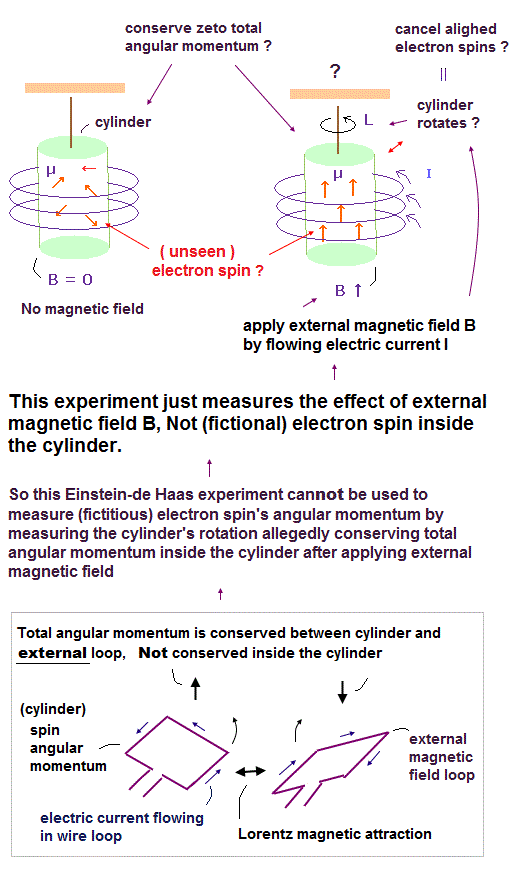It is said that Einstein-de Haas effects showed "Spin" means "actual rotation".
But if spin is rotation, its spinning speed must be much faster than light speed, as I said in this section.
This is strange.

In Fig.50 left, the magnetic field B in the iron cylinder is zero, and the magnetic dipole moment vectors (= μ ) of its atoms are randomly oriented.
In Fig.50 right, when a magnetic field is set up along the cylinder's axis, the μ line up parallel to and the cylinder begins to rotate. See this site p.4 or this.

Some physicists at that time wrongly thought when atomic spins inside cylinder change its direction of spin (= angular momentum ) by external magnetic field of coils, other atoms inside the cylinder may rotate in the opposite direction of atomic spins to conserve total angular momentum inside cylinder.

↑ This idea of total angular momentum conservation inside cylinder is wrong due to the existence of external magnetic force (= For the total angular momentum inside cylinder to be conserved, Only atoms inside the cylinder have to influence each other without external magnetic force ).

Because atomic spins inside the cylinder were changed by external (= Not internally ) magnetic force from the electric current of the coils, hence, the total angular momentum inside the cylinder is Not conserved (= because external force changes their spin angular momentum direction )

The wrong interpretation of this experiment came from physicists having No way to figure out the realistic mechanism or image of how unrealistic electron spins interact with external electromagnetic fields when changing its directions.

Some people say this experiments proved spin g factor = "2".
But this experiment is extremely difficult, and needs forcible interpretation.
( Even if each spin direction changes, the whole cylinder does NOT need to rotate ! ← Point. )
And the original Einstein experiment showed g factor is just "1" like classical orbit.

(Fig.51) Spin = "actual" rotation ?? → speed > 100 c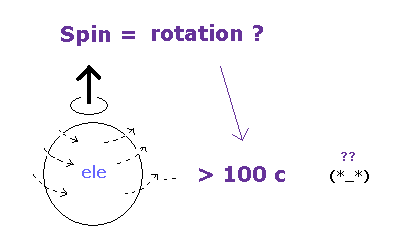On page 78-79 in the book, "Einstein's unification", by Jeroen van Dongen,
----------------------------------------
Einstein and de Haas's first measurements gave results that were equivalent to g = 1.4. It was soon clear that a systematic error had crept in: the magnetic field used had been too weak. A second, improved measurement gave a more satisfactory result: g = 1.02 +- 0.10. ( ← classical orbit ! )
Einstein and de Haas concluded that Ampere's hypothesis had been fairly confirmed by our observations.

The discovery of electron-spin, in 1925, could also give a theoretical explanation of that value ( g = "2" ).
Why Einstein and de Haas measure a value g (= 1 ) ?
Their experimental arrangement in all likelihood still allowed for too many systematic errors. The earth's magnetic field, for instance, was a disturbing factor.
------------------------------------------
As you see, the original Einstein de Haas's experiment showed g factor is "1" like classical orbit.
And this experiment is extremely difficult, susceptible to earth magnetic field. See also this site.

[ Electrons inside "coil" ( NOT fiber ) and cylinder are linked. ]

(Fig.52) Spin angular momentum can really rotate the "whole" cylinder ?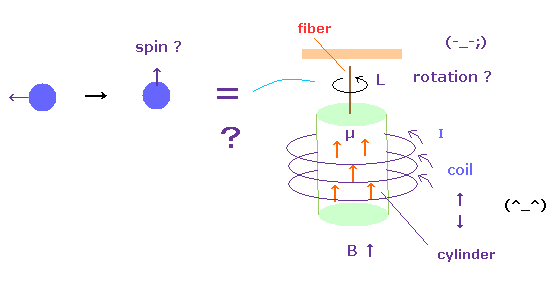The problem is that the change of each spin angular momentum can really rotate the whole iron cylinder ?
Of course, each electron ( or proton ) is separated from each other.
This means the magnetic field acts as some force to change each angular momentum.
This magnetic field can transmit through vacuum, so they can influence many atoms inside coil.

It is natural that we think their angular momentums (= magnetic moments ) are mainly transmitted to atoms inside coils rather than fiber.
Because depending on electrons' motions (= current I ) inside coil, the directions of spin in the cylinder change. Or imagine Faraday law. So electrons inside coil and cylinder are influencing each other.

And even if each electron spin becomes parallel to each other, the whole sylinder does NOT need to rotate, unless protons are influenced.
( For example, ferromagnets such as iron are NOT rotating. )
So the interpretation of these experiments are very artificial, and cannot be accepted as it is.2013/12/26 updated. Feel free to link to this site.